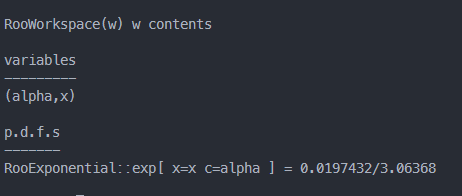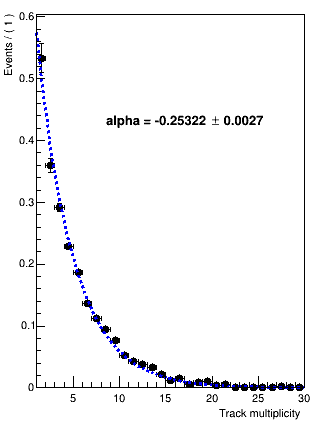Hi! I am use print to show what I store RooWorkspace, the result show in belowI want to ask is anyone know what is the 0.0197432 meaning ?

Here is my roofit code

``````void makeModel(RooWorkspace &w)
{
w.factory("Exponential::exp(x[1,30], alpha[-1.54,-1., 1.0])");
}
void DY_fit()
{
TFile *file = new TFile("./../ee_DY_emjet_half.root");
TH1F *h_fake_rate = ((TH1F *)file->Get("HT_bjet_fakeRate"));
RooRealVar x("x", "Track multiplicity ", 1, 30);
RooDataHist data("data", "dataset with x", x, h_fake_rate);
RooWorkspace w("w");
makeModel(w);
w.pdf("exp")->chi2FitTo(data, Save());
TCanvas *c1 = new TCanvas();
RooPlot *xframe = x.frame(Title("DY bjet fakeRate"));
data.plotOn(xframe);
w.pdf("exp")->plotOn(xframe, LineStyle(kDashed));
w.pdf("exp")->paramOn(xframe, Layout(0.3, 0.5, 0.7));
cout << "chi^2 = " << xframe->chiSquare() << endl;
cout << "normalization factor = " << 1 / w.pdf("exp")->getNorm(RooArgSet(x)) << endl;
RooHist *hpull = xframe->pullHist();
c1->Divide(2, 1);
c1->cd(1);
xframe->Draw();
c1->cd(2);
hpull->Draw();
w.Print();
w.writeToFile("model.root");
}
``````

and here is the my roofit result_ROOT Version: ROOT 6.26/00
_Platform: RooFit
_Compiler: Ubuntu